## Stirling's Approximation

Stirling's approximation gives an approximate value for the Factorial function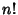or the Gamma Functionfor. The approximation can most simply be derived foran Integer by approximating the sum over the terms of the Factorial with an Integral, so that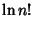(1)

The equation can also be derived using the integral definition of the Factorial,(2)

Note that the derivative of the Logarithm of the integrand can be written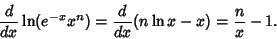(3)

The integrand is sharply peaked with the contribution important only near. Therefore, letwhere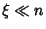, and write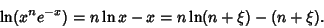(4)

Now,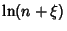(5)

so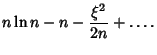(6)

Taking the Exponential of each side then gives(7)

Plugging into the integral expression forthen gives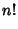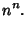(8)

Evaluating the integral gives(9)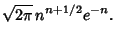(10)

Taking the Logarithm of both sides then gives(11)

This is Stirling's Series with only the first term retained and, for large, it reduces to Stirling's approximation(12)

Gosper notes that a better approximation to(i.e., one which approximates the terms in Stirling's Series instead of truncating them) is given by(13)

This also gives a much closer approximation to the Factorial of 0,, yieldinginstead of 0 obtained with the conventional Stirling approximation.